Function Repository Resource:

# TwistedSurface

Generate a surface twisting a curve

Contributed by: Wolfram Staff (original content by Alfred Gray)
 ResourceFunction["TwistedSurface"][c,a,b,{u,v}] gives the parametric surface parameterized by variables u and v and generated by a generatrix curve c at a distance a from the origin, twisted b times.

## Details and Options

Twisted surfaces are a class of surfaces generated by torsion. "Twisting" effectively rotates the curve around the z-axis.
ResourceFunction["TwistedSurface"] generalizes the construction of the Möbius strip and Klein bottle, which are special cases.
The profile curve c must be a plane curve with the property that c(-t)=-c(t) where c(t)=(φ(t),ψ(t)). The corresponding twisted surface is defined by (a+cos(b u)φ(v)-sin(b u)ψ(v))(cos(u),sin(u),0)+sin(b u)ψ(v)+cos(b u)ψ(v))(0,0,1).

## Examples

### Basic Examples (2)

The Möbius strip is generated with a half twist:

 In:=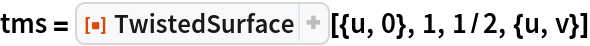Out=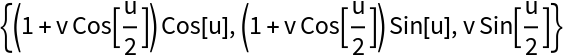In:=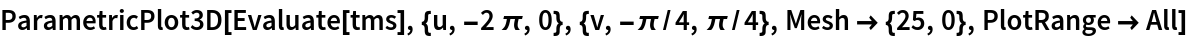Out=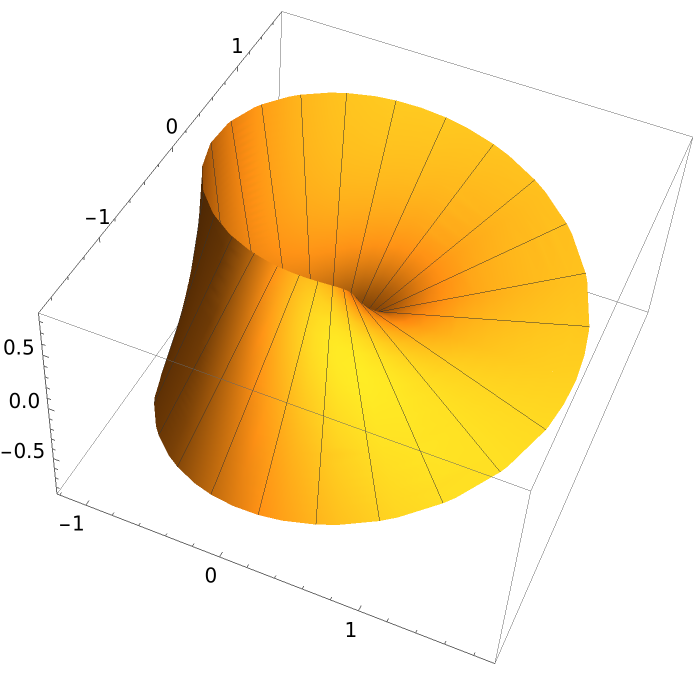See how the surface is generated:

 In:=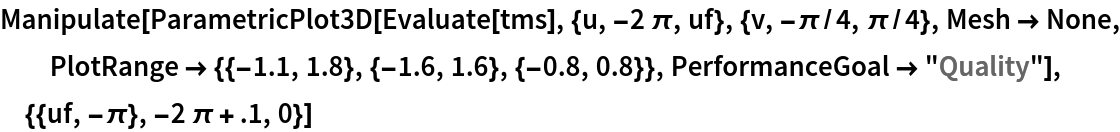Out=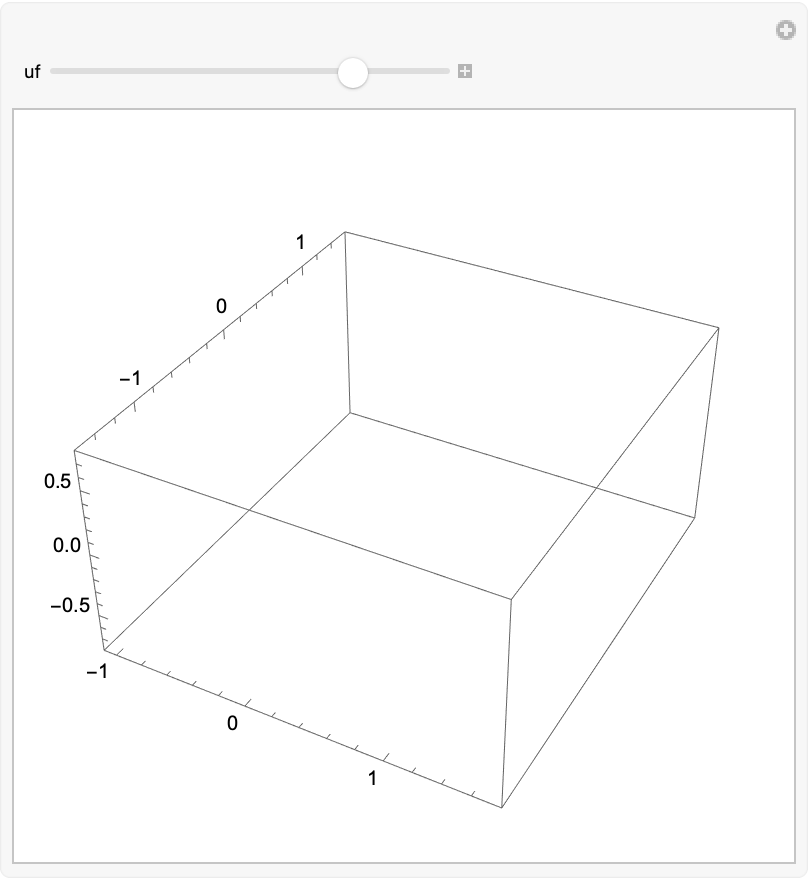### Scope (4)

Increase the distance from the origin:

 In:=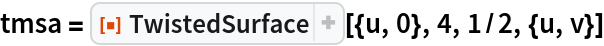Out=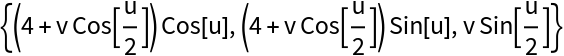In:=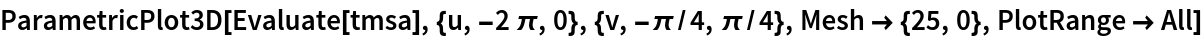Out=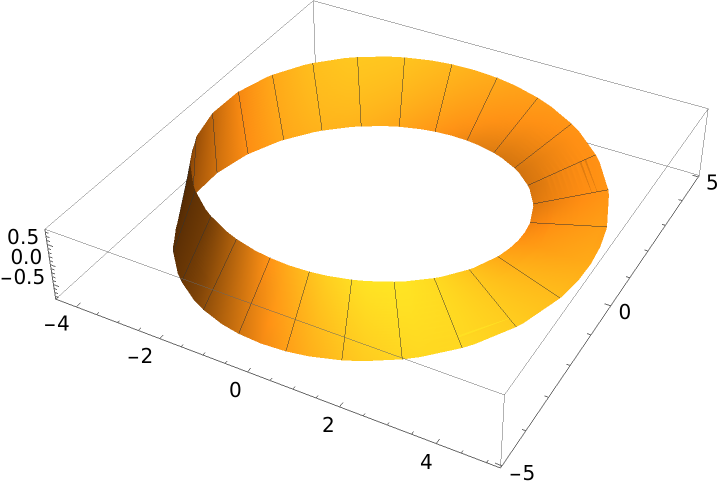A complete twist:

 In:=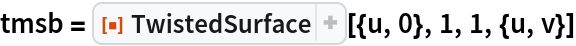Out=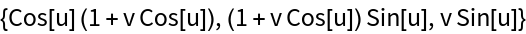In:=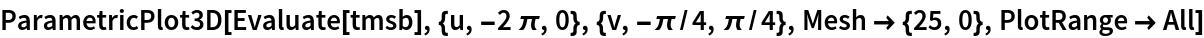Out=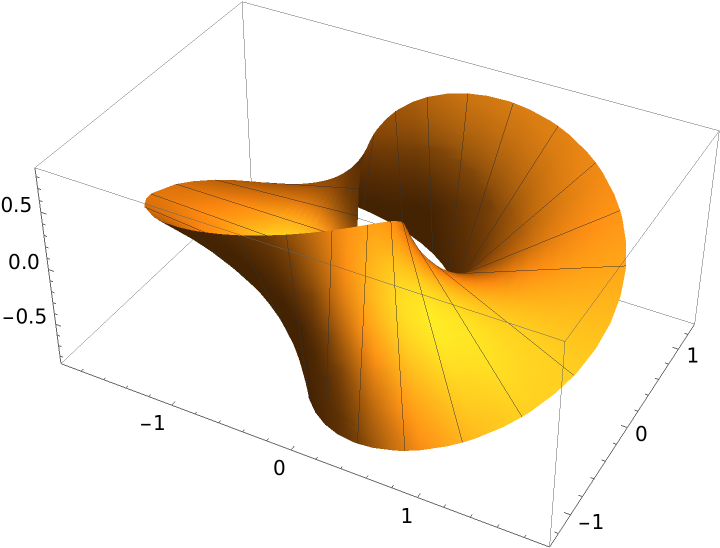A lemniscate:

 In:=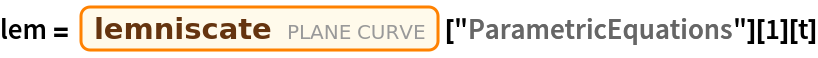Out=A twist of a lemniscate gives the figure–eight parametrization of a Klein bottle:

 In:=Out=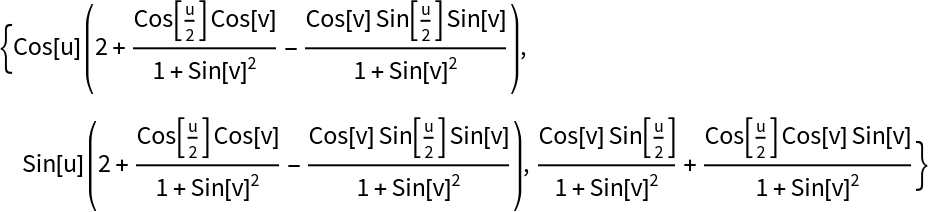Plot the Klein bottle:

 In:=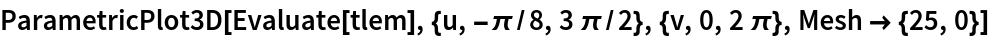Out=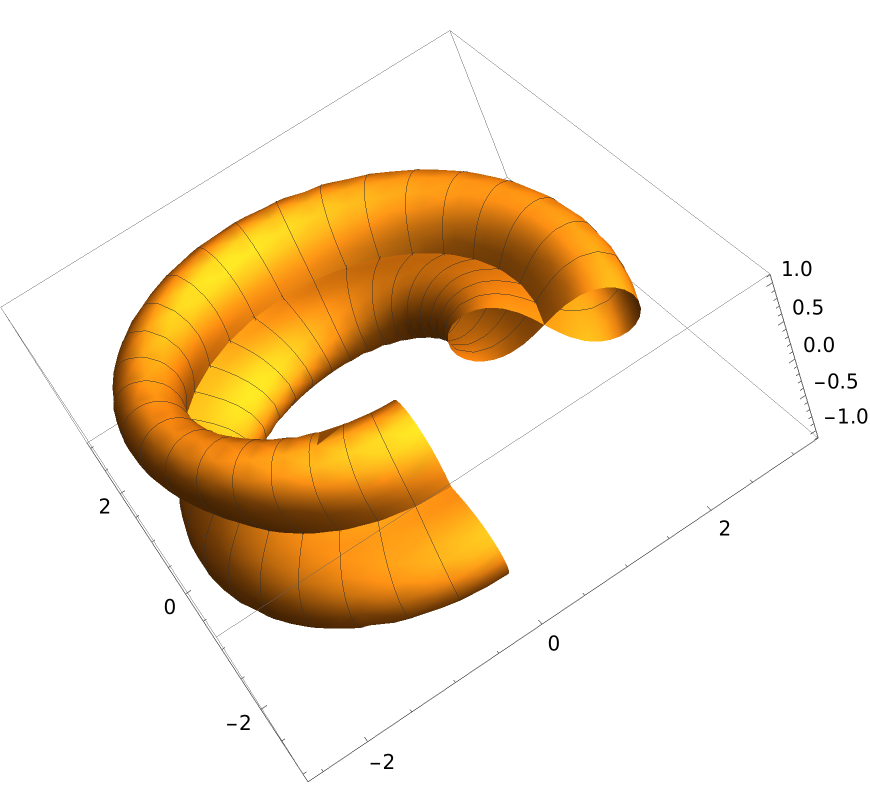A Lissajous curve:

 In:=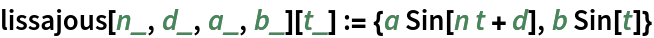A twist of the Lissajous curve:

 In:=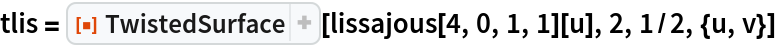Out=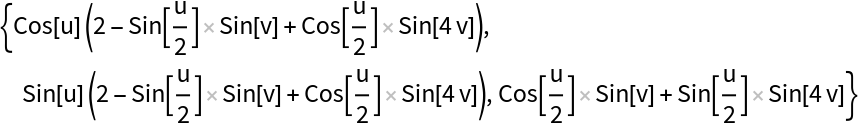Plot the resulting surface:

 In:=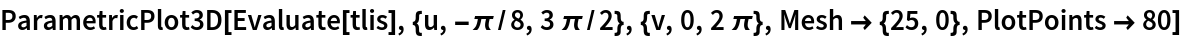Out=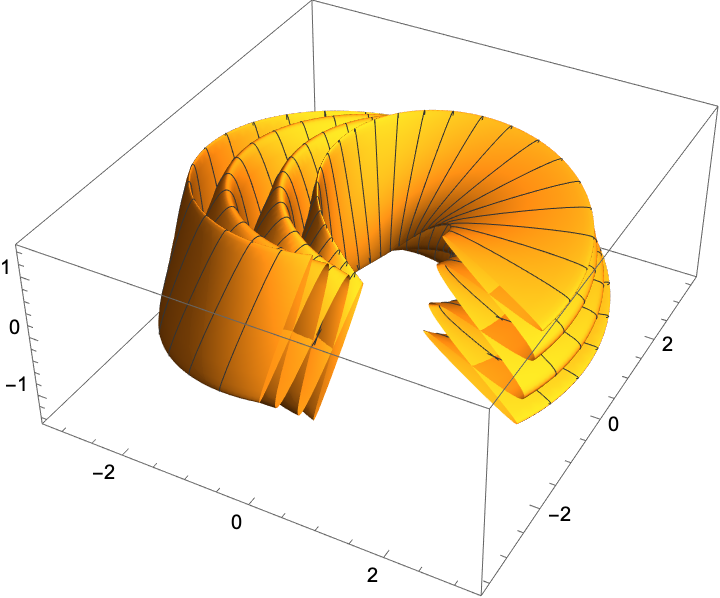Enrique Zeleny

## Version History

• 1.0.0 – 14 August 2020